### Chemical Reactions And Equations Class 10th Science CBSE Solution

##### Question 1.Which of the statements about the reaction below are incorrect?2Pb0 (s) + C (s) → 2Pb (s) + CO2(g)(a) Lead is getting reduced(b) Carbon dioxide is getting oxidised(c) Carbon is getting oxidised(d) Lead oxide is getting reducedA. (a) and (b)B. (a) and (c)C. (a), (b) and (c)D. allAnswer:Loss of Oxygen is by an element is known Reduction whereas gain of Oxygen by an element is known as OxidationHere, lead oxide loses oxygen and is hence reduced. Carbon gains oxygen and hence gets oxidized.Question 2.Fe203 + 2Al → Al203 + 2FeThe above reaction is an example of a:A. combination reactionB. double displacement reactionC. decomposition reactionD. displacement reactionAnswer:When an element displaces another element from its compound, a displacement reaction occurs.In this reaction, aluminium displaces the iron from its compound. Thus, this is an example of displacement reaction.Question 3.What happens when dilute hydrochloric acid is added to iron filings? Tick the correct answer.A. Hydrogen gas and iron chloride are producedB. Chlorine gas and iron hydroxide are producedC. No reaction takes placeD. Iron salt and water are producedAnswer:When dilute hydrochloric acid is added to iron fillings, hydrogen gas and iron chloride are produced.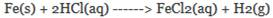Question 4.What is a balanced chemical equation? Why should chemical equations be balanced?Answer:A chemical reaction which has an equal number of atoms of the elements in the reactants and product sides is called a balanced chemical equation.For example;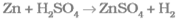Since the number of atoms of each element is the same on both parts of the equation. Hence, it is a balanced chemical equation.The chemical equation needs to be balanced so that it follows the law of conservation of mass(which states that the 'matter (or atoms) can neither be created nor destroyed in a chemical reaction')Question 5.Translate the following statements into chemical equations and then balance them:(a) Hydrogen gas combines with nitrogen to form ammonia.(b) Hydrogen sulphide gas burns in air to give water and sulphur dioxide.(c) Barium chloride reacts with aluminium sulphate to give aluminium chloride solution and a precipitate of barium sulphate.(d) Potassium metal reacts with water to give potassium hydroxide and hydrogenAnswer:(a) Hydrogen gas combines with nitrogen to form ammonia.The equation of the above reaction isH2 (g) + N2 (g) → NH3 (g)Step 1 - To balance chemical equations, first list the number of atoms of a different type that are present in the chemical equation on both sides –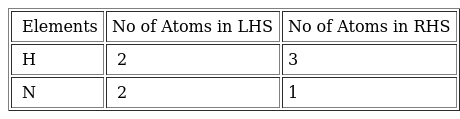Step 2 - To start balancing, we take all compound in the reaction and start balancing the reaction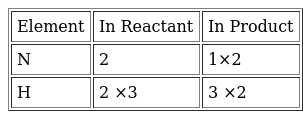Hence the Balanced Chemical Equation is 3H2 + N2 →2NH3The following questions are solved similarly.(b)Hydrogen sulphide gas burns in air to give water and sulphur dioxide.The equation of the above chemical change isH2S + O2 → H2O + SO2Step 1 - To balance chemical equations, first list the number of atoms of a different type that are present in the chemical equation on both sides –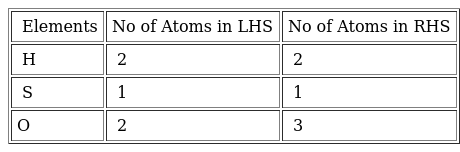Step 2 - To start balancing, we take all compound in the reaction and start balancing the reaction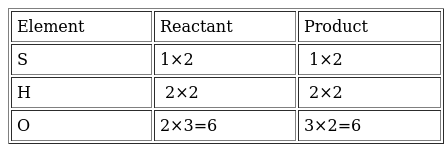Hence, the Balanced Chemical Equation is2H2S (g) + 3O2 (g) → 2H2O (l) + 2SO2 (g)The following questions are solved similarly(c) Barium chloride reacts with aluminium sulphate to give aluminium chloride solution and a precipitate of barium sulphate.The equation of the above reaction isBaCl2 + Al2(SO4)3 (aq) → AlCl3 (aq) +BaSO4 (s)Step 1 - To balance chemical equations, first list the number of atoms of a different type that are present in the chemical equation on both sides –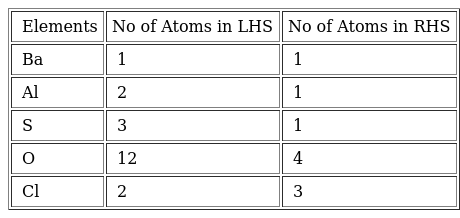Step 2 - To start balancing, we take all compound in the reaction and start balancing the reaction.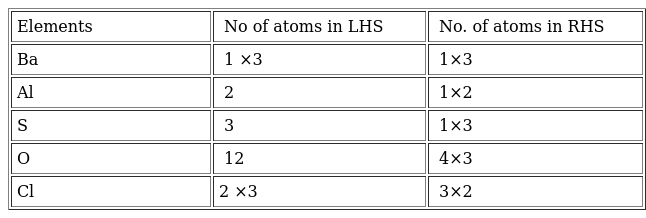Thus, we get3BaCl2 (aq) + Al2(SO4)3 (aq) → 2AlCl3 (aq) + 3BaSO4 (s)(d) Potassium metal reacts with water to give potassium hydroxide and hydrogenThe equation of the above reaction isK + H2O → KOH +H2Step 1 - To balance chemical equations, first list the number of atoms of a different type that are present in the chemical equation on both sides –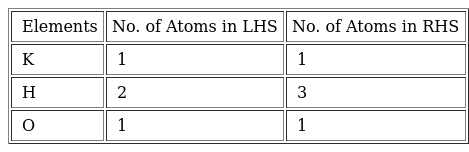Step 2 - To start balancing, we take all compound in the reaction and start balancing the reaction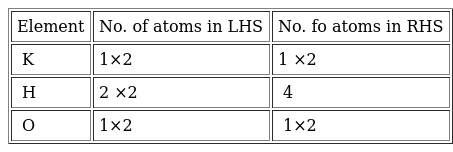Thus, using the above table the balanced chemical equation is2K (s) + 2H2O (l) → 2KOH (aq) + H2 (g)Question 6.Balance the following chemical equations:(a) HNO3 + Ca(OH)2→ Ca(NO3)2 + H2O(b) NaOH + H2SO4→ Na2SO4 + H2O(c) NaCl + AgNO3→ AgCl + NaNO3(d) BaCl2 + H2SO4→ BaSO4 + 2HCLAnswer:(a) Step 1 - To balance chemical equations, first list the number of atoms of different type that are present in the chemical equation on both sides -HNO3 + Ca(OH)2→ Ca(NO3)2 + H2O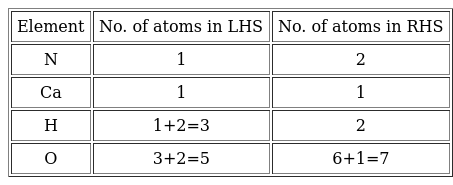Step 2 - To start balancing, we find the compound that has the highest number of atoms. We can see that Ca(NO3)2 on the product side has the highest number of atoms. In this compound Oxygen has the highest number of atoms. Lets start with that.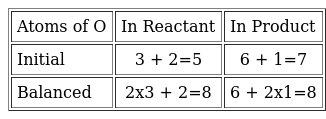This makes the partially balanced equation -2HNO3 + Ca(OH)2→ Ca(NO3)2 + 2H2OStep 3 - Now checking for all other elements we note that the number of atoms on both side are same. We can say that the equation is balanced. (b)NaOH + H2SO4→ Na2SO4 + H2OStep 1 - To balance chemical equations, first list the number of atoms of different type that are present in the chemical equation on both sides –NaOH + H2SO4→ Na2SO4 + H2O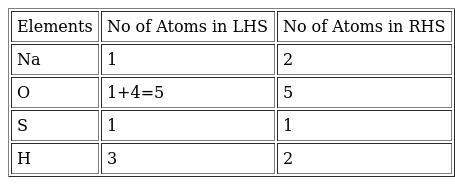Step 2 - To start balancing, we take any one compound. We will take NaOHon the reactant side in consideration. Let’s start with that.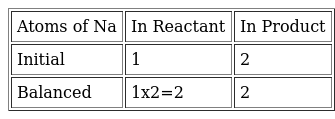This makes the partially Balanced Equation as:-2NaOH + H2SO4-> Na2SO4 + 2H2OStep 3 - Now checking for all other elements we note that the numbers of atoms on both sides are same. We can say that the equation is balanced. (c) NaCl + AgNO3→ AgCl + NaNO3Step 1 - To balance chemical equations, first list the number of atoms of different type that are present in the chemical equation on both sides –NaCl + AgNO3→ AgCl + NaNO3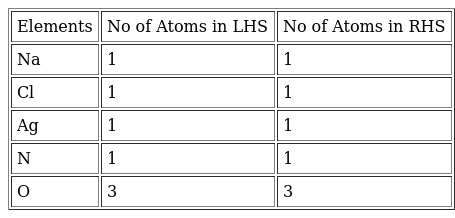We can see that the Number of Atoms in LHS and RHS are equal, Hence the equation is already balanced(d) BaCl2 + H2SO4→ BaSO4 + 2HCLStep 1 - To balance chemical equations, first list the number of atoms of different type that are present in the chemical equation on both sides –BaCl2 + H2SO4→ BaSO4 + 2HCL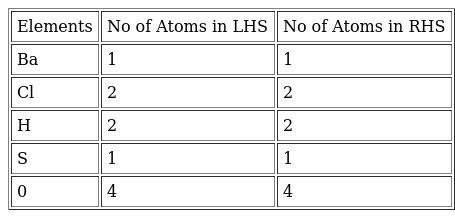We can see that the Number of Atoms in LHS and RHS is equal, Hence the equation is already balanced. Question 7.Write the balanced chemical equations for the following reactions:(a) Calcium hydroxide + Carbon dioxide → Calcium carbonate + Water(b) Zinc + Silver nitrate → Zinc nitrate + Silver(c) Aluminium + Copper chloride → Aluminium chloride + Copper(d) Barium chloride + Potassium sulphate → Barium sulphate + Potassium chlorideAnswer:(a) Ca(OH)2 + CO2→ CaC03 + H20(b) Zn + 2AgNO3→ Zn(NO3)2 + 2Ag(c) 2Al + 3CuCl2→ 2AlCl3 + 3Cu(d) BaCl2 + K2SO4→ BaSO4 + 2KClQuestion 8.Write the balanced chemical equation for the following and identify the type of reaction in each case.(a) Potassium Bromide (aq) + Barium iodide (aq) →Potassium iodide (aq) +Barium bromide (s)(b) Zinc carbonate(s) → Zinc oxide(s) + Carbon dioxide (g)(c) Hydrogen(g) + Chlorine (g) → Hydrogen chloride (g)(d) Magnesium + Hydrochloric →Magnesium chloride (aq) + Hydrogen (g)Answer:(a) Double displacement reaction (also known as precipitation reaction):-2KBr (aq) + BaI2 (aq) → 2KI(q) + BaBr2 (s)(b) Decomposition reaction:ZnC03 (s) → ZnO (s) + C02 (g)(c) Combination reaction:H2 (g) + Cl2 (g) → 2HCl (g)(d) Displacement reaction:Mg (s) + 2HCl (aq) → MgCl2 (aq) + H2 (g) Question 9.What does one mean by exothermic and endothermic reactions? Give examples.Answer:Exothermic reaction: Reactions in which heat is released along with the formation of products are called exothermic reaction.For example: Burning of fuel is an example of exothermic reaction. When methane is burnt in the air, heat is produced along with carbon dioxide and water.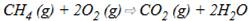Endothermic reaction: Reactions in which energy is absorbed are known as endothermic reactions.Decomposition reactions are example of endothermic reactions because they require energy in the form of heat, light or electricity for breaking down the reactants.Example: Sliver chloride turns grey in sunlight to form silver metal.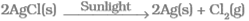Question 10.Why respiration is considered an exothermic reaction? Explain.Answer:In the process of respiration, the glucose combines with oxygen in the cells of our body to form carbon dioxide and energy is released. That’s why it is considered an exothermic reaction.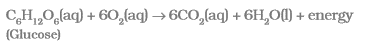Question 11.Why decomposition reactions are called the opposite of combination reactions? Write equations for these reactions.Answer:In a decomposition reaction, a single substance splits to form two or more simpler substances whereas in combination reaction, two or more substances combine to form a single substance. Hence, decomposition reactions are called the opposite of combination reactions.(i) Decomposition reaction: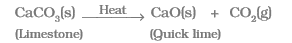In this reaction, calcium carbonate decomposes into calcium oxide and carbon dioxide on heating. This is an important decomposition reaction used in various industries.(ii) Combination reaction: Formation of water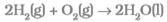In this reaction, two substances hydrogen and oxygen combine to form single substance water, so this is a combination reaction.We can see from the above examples that a decomposition reaction is opposite of a combination reaction.Question 12.Write one equation each for decomposition reactions where energy is supplied in the form of heat, light or electricity.Answer:(a) In this reaction, calcium carbonate decomposes into calcium oxide and carbon dioxide on heating. This reaction is carried out by heating.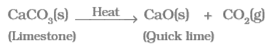In this reaction, energy is supplied in the form of heat.(b) Sliver chloride turns grey in sunlight to form silver metal.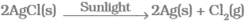In this reaction, energy is supplied in the form of light, i.e. sunlight.(c) When acidified water is electrolysed, it decomposes to form hydrogen and oxygen:2H20 (I) → 2H2 (g) + O2 (g)In this case, energy is supplied in the form of electricity.Question 13.What is the difference between displacement and double displacement reactions? Write equations for these reactions.Answer:In a displacement reaction, a more reactive element displaces a less reactive element from its solution while in a double displacement reaction, exchange of ions between the reactants takes place to form new products.(i) Displacement reaction: In this reaction, zinc displaced copper from copper sulphate solution.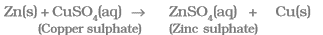(ii) Double displacement reaction: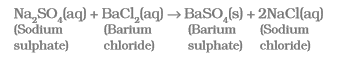Question 14.In the refining of silver, the recovery of silver from silver nitrate solution involved "displacement by copper metal. Write down the reaction involved.Answer:Displacement reaction is used in the recovery of silver from silver nitrate solution during refining of silver.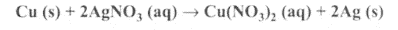Question 15.What do you mean by a precipitation reaction? Explain by giving example.Answer:Precipitation reaction: Any reaction in which an insoluble solid (called precipitate) is formed, is called a precipitation reaction.For example: When a solution of silver nitrate is mixed with a solution of sodium chloride, a white precipitate of silver chloride is formed. Hence, it is precipitation reaction.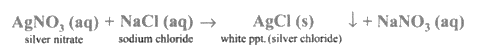Question 16.Explain the following in terms of gain or loss of oxygen with two examples each:(a) Oxidation (b) ReductionAnswer:(a) Oxidation: A chemical reaction in which gain of oxygen or loss of hydrogen takes place.Example: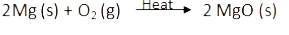Here, magnesium is oxidised to form magnesium oxide.(b) Reduction: A chemical reaction in which loss of oxygen or gain of hydrogen takes place.Example: When zinc oxide is heated with carbon, then zinc metal and carbon monoxide are formed: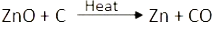In this reaction, Zinc oxide (ZnO) is reduced to zinc by the loss of oxygen.Question 17.A shiny brown coloured element X on heating in the air becomes black in colour. Name the element X and the black coloured compound formed.Answer:The unknown element X is copper (Cu). When copper is heated in air, it forms a black coloured compound called copper oxide (CuO). The reaction for above chemical change is given below: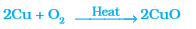The color of the heated copper powder becomes black when air is passed over it because of its reaction with oxygen i.e Air. When it reacts with oxygen it forms copper oxide which is black in color. Question 18.Why do we apply paint on iron articles?Answer:Iron articles are painted to prevent them from rusting. When paint is applied on the surface of iron articles, the contact of air and moisture with iron metals is cut off. Therefore, no rusting takes place.Question 19.Oil and fat containing food items are flushed with nitrogen. Why?Answer:The oil and fat present in food items get oxidized in the presence of air forming products having unpleasant smell and taste which turn the food rancid.Rancidity makes the food unfit for eating.This is why antioxidants are added to foods containing oil and fat to prevent oxidation of food.Nitrogen acts as an antioxidant.That’s why oil and fat containing food items are flushed with nitrogen to prevent oxidation.Thus, the food does not turn rancid and remain good to eat for a longer time.Question 20.Explain the following terms with one example each: (a) Corrosion (b) RancidityAnswer: (a) Corrosion: Gradual Deterioration of metals by the action of :-1.Air 2.Moisture 3.Chemical( such as an acid) on their surface. Main Cause of corrosion is the oxidation of metals by the oxygen of air.Example:- Rusting of Iron - When a piece of Iron is left out in damp time for a period of time it gets covered with a red-brown substance called rust.The following reaction takes place resulting in then formation of rust:-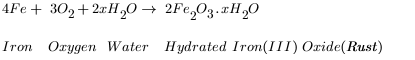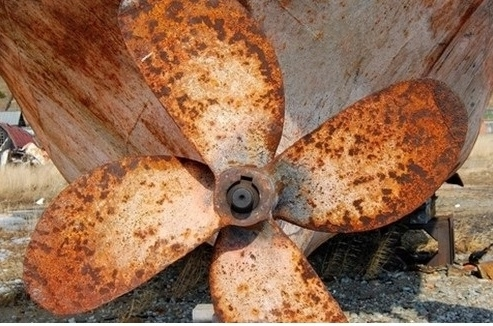(b) Rancidity:- Oxidation of Fats and Oils present in food materials by the oxygen present in air resulting in unpleasant smell and taste is called Rancidity. This makes the food not fit for eating.Example:- Potato Chips that are cooked in oil turn Rancid and give out unpleasant smell and taste if kept exposed for a long time

PDF FILE TO YOUR EMAIL IMMEDIATELY PURCHASE NOTES & PAPER SOLUTION. @ Rs. 50/- each (GST extra)

HINDI ENTIRE PAPER SOLUTION

MARATHI PAPER SOLUTION

SSC MATHS I PAPER SOLUTION

SSC MATHS II PAPER SOLUTION

SSC SCIENCE I PAPER SOLUTION

SSC SCIENCE II PAPER SOLUTION

SSC ENGLISH PAPER SOLUTION

SSC & HSC ENGLISH WRITING SKILL

HSC ACCOUNTS NOTES

HSC OCM NOTES

HSC ECONOMICS NOTES

HSC SECRETARIAL PRACTICE NOTES

# 2019 Board Paper Solution

HSC ENGLISH SET A 2019 21st February, 2019

HSC ENGLISH SET B 2019 21st February, 2019

HSC ENGLISH SET C 2019 21st February, 2019

HSC ENGLISH SET D 2019 21st February, 2019

SECRETARIAL PRACTICE (S.P) 2019 25th February, 2019

HSC XII PHYSICS 2019 25th February, 2019

CHEMISTRY XII HSC SOLUTION 27th, February, 2019

OCM PAPER SOLUTION 2019 27th, February, 2019

HSC MATHS PAPER SOLUTION COMMERCE, 2nd March, 2019

HSC MATHS PAPER SOLUTION SCIENCE 2nd, March, 2019

SSC ENGLISH STD 10 5TH MARCH, 2019.

HSC XII ACCOUNTS 2019 6th March, 2019

HSC XII BIOLOGY 2019 6TH March, 2019

HSC XII ECONOMICS 9Th March 2019

SSC Maths I March 2019 Solution 10th Standard11th, March, 2019

SSC MATHS II MARCH 2019 SOLUTION 10TH STD.13th March, 2019

SSC SCIENCE I MARCH 2019 SOLUTION 10TH STD. 15th March, 2019.

SSC SCIENCE II MARCH 2019 SOLUTION 10TH STD. 18th March, 2019.

SSC SOCIAL SCIENCE I MARCH 2019 SOLUTION20th March, 2019

SSC SOCIAL SCIENCE II MARCH 2019 SOLUTION, 22nd March, 2019

XII CBSE - BOARD - MARCH - 2019 ENGLISH - QP + SOLUTIONS, 2nd March, 2019

# HSCMaharashtraBoardPapers2020

(Std 12th English Medium)

HSC ECONOMICS MARCH 2020

HSC OCM MARCH 2020

HSC ACCOUNTS MARCH 2020

HSC S.P. MARCH 2020

HSC ENGLISH MARCH 2020

HSC HINDI MARCH 2020

HSC MARATHI MARCH 2020

HSC MATHS MARCH 2020

# SSCMaharashtraBoardPapers2020

(Std 10th English Medium)

English MARCH 2020

HindI MARCH 2020

Hindi (Composite) MARCH 2020

Marathi MARCH 2020

Mathematics (Paper 1) MARCH 2020

Mathematics (Paper 2) MARCH 2020

Sanskrit MARCH 2020

Sanskrit (Composite) MARCH 2020

Science (Paper 1) MARCH 2020

Science (Paper 2)

Geography Model Set 1 2020-2021

MUST REMEMBER THINGS on the day of Exam

Are you prepared? for English Grammar in Board Exam.

Paper Presentation In Board Exam

How to Score Good Marks in SSC Board Exams

Tips To Score More Than 90% Marks In 12th Board Exam

How to write English exams?

How to prepare for board exam when less time is left

How to memorise what you learn for board exam

No. 1 Simple Hack, you can try out, in preparing for Board Exam

How to Study for CBSE Class 10 Board Exams Subject Wise Tips?

JEE Main 2020 Registration Process – Exam Pattern & Important Dates

NEET UG 2020 Registration Process Exam Pattern & Important Dates

How can One Prepare for two Competitive Exams at the same time?

8 Proven Tips to Handle Anxiety before Exams!